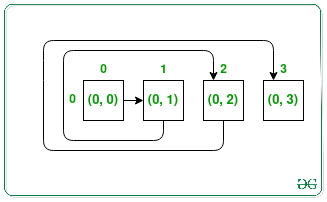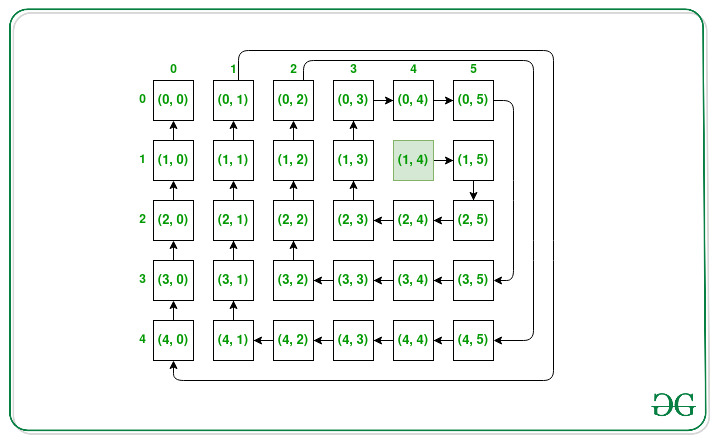Matrix Spiral Traversal starting from given Coordinates

• Difficulty Level : Expert
• Last Updated : 10 Jan, 2022

Given the order of the matrix N and M, and a source location (X, Y), the task is to find all the coordinates of the matrix in order when visited in clockwise spiral order((i.e. East-> South-> West-> North). Whenever moved outside the matrix, continue the walk outside it to return to the matrix later.

Examples:

Input: N = 1, M = 4, X = 0, Y = 0
Output: {{0, 0}, {0, 1}, {0, 2}, {0, 3}}
Explanation:Input: N = 5, M = 6, X = 1, Y = 4
Output: {{1, 4}, {1, 5}, {2, 5}, {2, 4}, {2, 3}, {1, 3}, {0, 3}, {0, 4}, {0, 5}, {3, 5}, {3, 4}, {3, 3}, {3, 2}, {2, 2}, {1, 2}, {0, 2}, {4, 5}, {4, 4}, {4, 3}, {4, 2}, {4, 1}, {3, 1}, {2, 1}, {1, 1}, {0, 1}, {4, 0}, {3, 0}, {2, 0}, {1, 0}, {0, 0}}
Explanation:Approach: The given problem can be solved by traversing the matrix from the position (X, Y) in a clockwise spiral direction, and whenever reached outside the matrix, don’t include the coordinates in the solution but continue traversing outside to reach again inside the matrix. Continue this process until all cells of the matrix are not included in the solution or result.

Below is the implementation of the above approach:

C++

 // C++ program for the above approach   #include using namespace std;   // Function to turn one unit left void turn_left(int& r, int& c) {     c -= 1; }   // Function to turn one unit right void turn_right(int& r, int& c) {     c += 1; }   // Function to turn one unit up void turn_up(int& r, int& c) {     r -= 1; }   // Function to turn one unit down void turn_down(int& r, int& c) {     r += 1; }   // For checking whether a cell lies // outside the matrix or not bool is_outside(int row, int col,                 int r, int c) {     if (r >= row || c >= col         || r < 0 || c < 0)         return true;     return false; }   // Function to rotate in clockwise manner void next_turn(char& previous_direction,                int& r, int& c) {     if (previous_direction == 'u') {         turn_right(r, c);         previous_direction = 'r';     }     else if (previous_direction == 'r') {         turn_down(r, c);         previous_direction = 'd';     }     else if (previous_direction == 'd') {         turn_left(r, c);         previous_direction = 'l';     }     else if (previous_direction == 'l') {         turn_up(r, c);         previous_direction = 'u';     } }   // Function to move in the same direction // as its prev_direction void move_in_same_direction(     char previous_direction,     int& r, int& c) {     if (previous_direction == 'r')         c++;     else if (previous_direction == 'u')         r--;     else if (previous_direction == 'd')         r++;     else if (previous_direction == 'l')         c--; }   // Function to find the spiral order of // of matrix according to given rules vector > spiralMatrixIII(     int rows, int cols, int r, int c) {     // For storing the co-ordinates     vector > res;     char previous_direction = 'r';       // For keeping track of no of steps     // to go without turn     int turning_elements = 2;       // Count is for counting total cells     // put in the res     int count = 0;       // Current_count is for keeping track     // of how many cells need to     // traversed in the same direction     int current_count = 0;       // For keeping track the number     // of turns we have made     int turn_count = 0;     int limit = rows * cols;       while (count < limit) {           // If the current cell is within         // the board         if (!is_outside(rows, cols, r, c)) {             res.push_back({ r, c });             count++;         }         current_count++;           // After visiting turning elements         // of cells we change our turn         if (current_count == turning_elements) {               // Changing our direction             // we have to increase the             // turn count             turn_count++;               // In Every 2nd turn increasing             // the elements the turn visiting             if (turn_count == 2)                 turning_elements++;               // After every 3rd turn reset             // the turn_count to 1             else if (turn_count == 3) {                 turn_count = 1;             }               // Changing direction to next             // direction based on the             // previous direction             next_turn(previous_direction, r, c);               // Reset the current_count             current_count = 1;         }         else {             move_in_same_direction(                 previous_direction, r, c);         }     }       // Return the traversal     return res; }   // Driver Code int main() {     int N = 5, M = 6, X = 1, Y = 4;     auto res = spiralMatrixIII(N, M, X, Y);       // Display answer     for (auto it : res) {         cout << '(' << it << ", "              << it << ')' << ' ';     }     return 0; }

Java

 // Java program for the above approach import java.io.*; import java.util.*; class GFG {         // For checking whether a cell lies     // outside the matrix or not     static Boolean is_outside(int row, int col, int r,                               int c)     {         if (r >= row || c >= col || r < 0 || c < 0) {             return true;         }         return false;     }       // Function to rotate in clockwise manner     static int[] next_turn(char previous_direction, int r,                            int c)     {         if (previous_direction == 'u') {             // turn_right             c += 1;             previous_direction = 'r';         }         else if (previous_direction == 'r') {             // turn_down             r += 1;             previous_direction = 'd';         }         else if (previous_direction == 'd') {             // turn_left             c -= 1;             previous_direction = 'l';         }         else if (previous_direction == 'l') {             // turn_up             r -= 1;             previous_direction = 'u';         }         int[] v = { previous_direction, r, c };         return v;     }       // Function to move in the same direction     // as its prev_direction     static int[] move_in_same_direction(         char previous_direction, int r, int c)     {         if (previous_direction == 'r')             c += 1;         else if (previous_direction == 'u')             r -= 1;         else if (previous_direction == 'd')             r += 1;         else if (previous_direction == 'l')             c -= 1;           int[] v = { r, c };         return v;     }         // Function to find the spiral order of     // of matrix according to given rules     static int[][] spiralMatrixIII(int rows, int cols,                                    int r, int c)     {         char previous_direction = 'r';           // For keeping track of no of steps         // to go without turn         int turning_elements = 2;           // Count is for counting total cells         // put in the res         int count = 0;           // Current_count is for keeping track         // of how many cells need to         // traversed in the same direction         int current_count = 0;           // For keeping track the number         // of turns we have made         int turn_count = 0;         int limit = rows * cols;           // For storing the co-ordinates         int[][] arr = new int[limit];           while (count < limit) {             // If the current cell is within             // the board               if (is_outside(rows, cols, r, c) == false) {                 arr[count] = r;                 arr[count] = c;                 count += 1;             }             current_count += 1;               // After visiting turning elements             // of cells we change our turn             if (current_count == turning_elements) {                   // Changing our direction                 // we have to increase the                 // turn count                 turn_count += 1;                   // In Every 2nd turn increasing                 // the elements the turn visiting                 if (turn_count == 2)                     turning_elements += 1;                   // After every 3rd turn reset                 // the turn_count to 1                 else if (turn_count == 3)                     turn_count = 1;                   // Changing direction to next                 // direction based on the                 // previous direction                 int[] store                     = next_turn(previous_direction, r, c);                   // converting integer back to Character                 previous_direction = (char)store;                 r = store;                 c = store;                 // Reset the current_count                 current_count = 1;             }             else {                 int[] store = move_in_same_direction(                     previous_direction, r, c);                 r = store;                 c = store;             }             // Return the traversal         }         return arr;     }         // Driver Code     public static void main(String[] args)     {         int N = 5;         int M = 6;         int X = 1;         int Y = 4;         int[][] arr = spiralMatrixIII(N, M, X, Y);         String ans = "";         int len = arr.length;         for (int i = 0; i < len; i++) {             ans += "(" + arr[i] + "," + arr[i] + ") ";         }         System.out.println(ans);     } }   // This code is contributed by rj13to.

Python3

 # Python program for the above approach   # For checking whether a cell lies # outside the matrix or not def is_outside(row, col, r, c):     if (r >= row or c >= col or r < 0 or c < 0):         return True     return False   # Function to rotate in clockwise manner def next_turn(previous_direction,               r, c):     if (previous_direction == 'u'):                 # turn_right         c += 1         previous_direction = 'r'     elif (previous_direction == 'r'):                 # turn_down         r += 1         previous_direction = 'd'     elif (previous_direction == 'd'):                 # turn_left         c -= 1         previous_direction = 'l'     elif (previous_direction == 'l'):                 # turn_up         r -= 1         previous_direction = 'u'     return [previous_direction, r, c]   # Function to move in the same direction # as its prev_direction def move_in_same_direction(         previous_direction, r, c):     if (previous_direction == 'r'):         c += 1     elif (previous_direction == 'u'):         r -= 1     elif(previous_direction == 'd'):         r += 1     elif(previous_direction == 'l'):         c -= 1     return [r, c]   # Function to find the spiral order of # of matrix according to given rules def spiralMatrixIII(rows, cols, r, c):     # For storing the co-ordinates     res = []     previous_direction = 'r'       # For keeping track of no of steps     # to go without turn     turning_elements = 2       # Count is for counting total cells     # put in the res     count = 0       # Current_count is for keeping track     # of how many cells need to     # traversed in the same direction     current_count = 0       # For keeping track the number     # of turns we have made     turn_count = 0     limit = rows * cols       while (count < limit):           # If the current cell is within         # the board         if (is_outside(rows, cols, r, c) == False):             res.append([r, c])             count += 1           current_count += 1           # After visiting turning elements         # of cells we change our turn         if (current_count == turning_elements):               # Changing our direction             # we have to increase the             # turn count             turn_count += 1               # In Every 2nd turn increasing             # the elements the turn visiting             if (turn_count == 2):                 turning_elements += 1               # After every 3rd turn reset             # the turn_count to 1             elif (turn_count == 3):                 turn_count = 1               # Changing direction to next             # direction based on the             # previous direction             store = next_turn(previous_direction, r, c)             previous_direction = store             r = store             c = store                           # Reset the current_count             current_count = 1           else:             store = move_in_same_direction(                 previous_direction, r, c)             r = store             c = store       # Return the traversal     return res   # Driver Code N = 5 M = 6 X = 1 Y = 4 res = spiralMatrixIII(N, M, X, Y)   # Display answer for vec in res:     print('(', vec, ", ", vec, ')', end=" ")       # This code is contributed by rj13to.

Output:
(1, 4) (1, 5) (2, 5) (2, 4) (2, 3) (1, 3) (0, 3) (0, 4) (0, 5) (3, 5) (3, 4) (3, 3) (3, 2) (2, 2) (1, 2) (0, 2) (4, 5) (4, 4) (4, 3) (4, 2) (4, 1) (3, 1) (2, 1) (1, 1) (0, 1) (4, 0) (3, 0) (2, 0) (1, 0) (0, 0)

Time Complexity: O(N2)
Auxiliary Space: O(1)

My Personal Notes arrow_drop_up
Recommended Articles
Page :